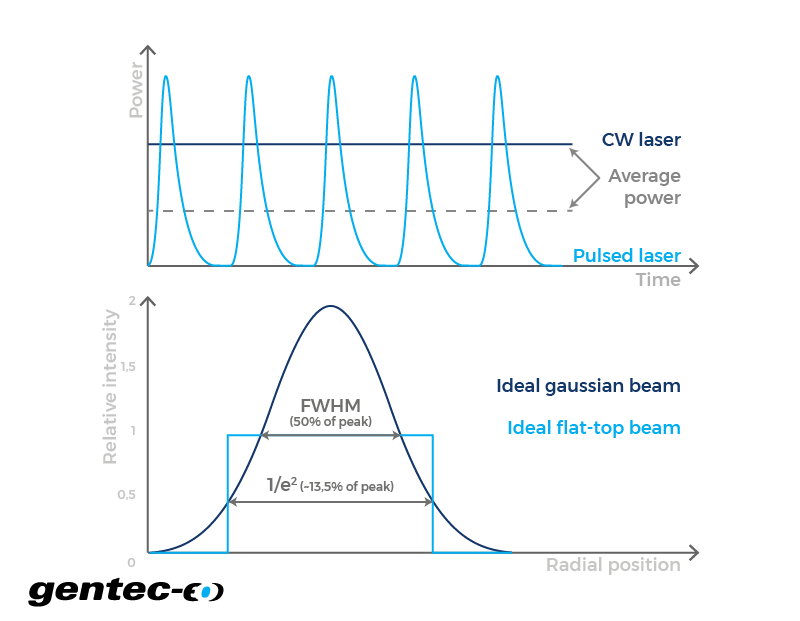EN

# Average power and power density

Convert laser average power and energy per pulse to average power density/irradiance and average power with this online calculator. Works for both Gaussian and flat beam profiles.

-

-

-

## How does the average power and power density calculator work?

The relation between average power, power density (irradiance) and beam size all comes down to one thing: what will happen to the surface at the receiving end of your laser over time. For a continuous wave laser, the average power is already known, but for a pulsed one, you need to multiply the energy in each pulse by the number of pulses by second. When the beam is shaped like a top-hat, the power is evenly distributed on the surface. This means that the power density is simply the average power divided by the size of the beam's cross-section. So, the power density is inversely proportional to the beam size. In a case where the power intensity distribution of a laser beam is described as a three-dimensional Gaussian function (Gaussian beam), its power density at the center is twice what you could expect from a circular top-hat shaped beam of diameter equal to 1/e². In both cases, the beam has a certain divergence (see our calculator about divergence). This implies that you can modulate the irradiance or average power density on a surface by moving it closer or farther away from the laser beam source.

Click to
enlarge## Formulas

The laser average power formulas describe the behavior of a theoretical flat-top or a perfect TEM₀₀ Gaussian laser beam. In such, they represent an approximation of the values one would obtain in real conditions. Also, there are multiple methods one can use to measure the diameter of a Gaussian beam. The reason for this mainly comes from the fact that its theoretical value only reaches 0 when the radius reaches infinity. Therefore, the beam would have an infinite diameter. So, we chose to use the method where it is measured using the 1/e² parameter. At this point, the beam diameter is approximately 1.699 times the full diameter measured at half the maximum of a Gaussian function (FWHM). At 1/e², it represents approximately 86,5% of the total power. Note that, for a flat-top beam, the equations are used just as is, but for a Gaussian beam, there is a factor 2 that multiplies the right hand part of these equations.

$$\text{Average power density} \left(\frac{W}{cm^2}\right) = \frac{\text{Average power}(W)}{\text{Beam area}(cm^2)}$$

Right-click
to copy

$$\text{Average power density} \left(\frac{W}{cm^2}\right) = \frac{\text{Energy per pulse}(J) \times \text{Repetition rate}(Hz)}{\text{Beam area}(cm^2)}$$

Right-click
to copy